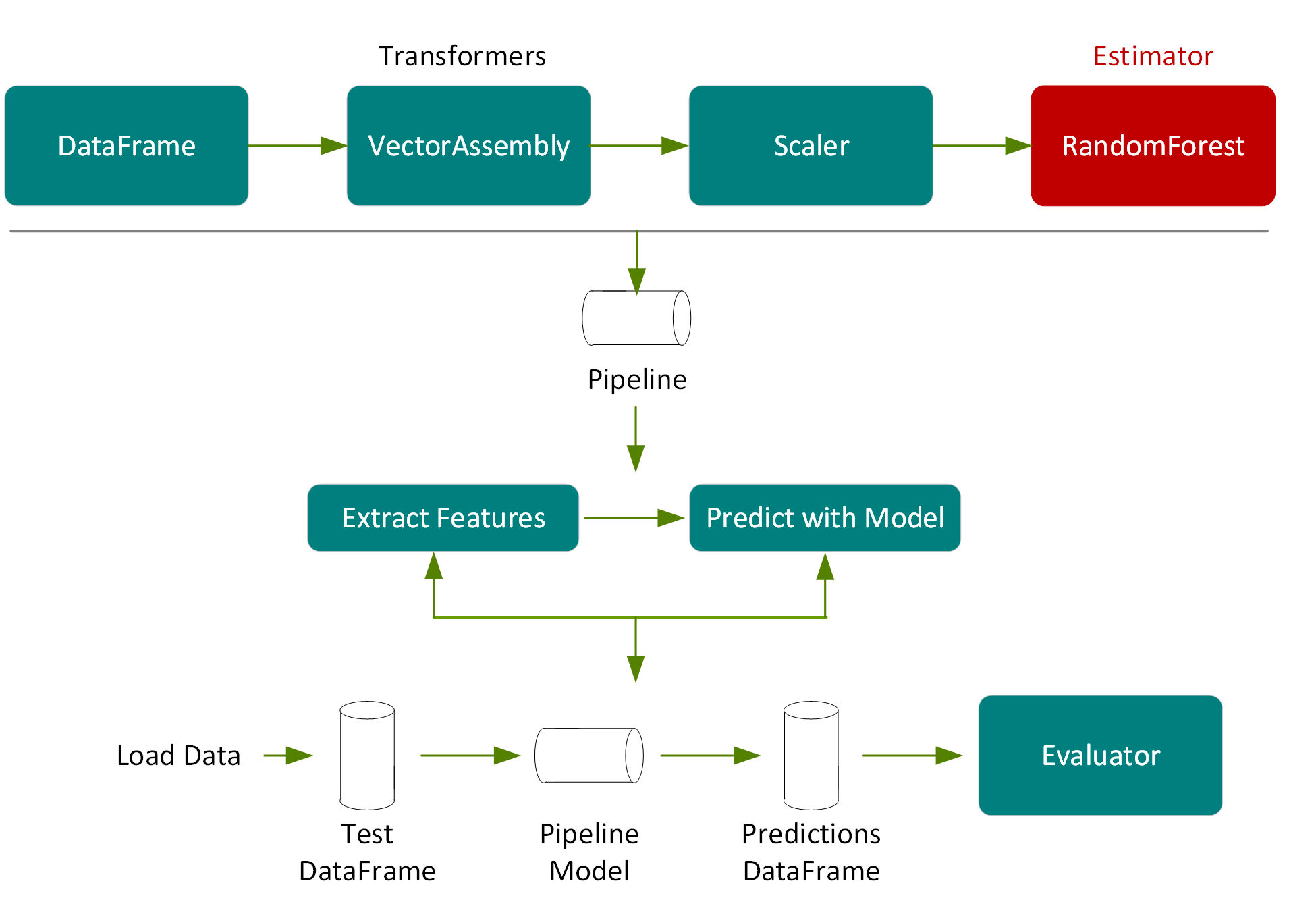# 使用 GPU 加速的 XGBoost 預測計程車車資

### XGBoost

XGBoost，代表極端梯度提升 (Extreme Gradient Boosting)，是種可擴充的分散式梯度提升決策樹 (GBDT) 機器學習函式庫。XGBoost 提供的平行決策樹提升，是處理迴歸、分類和排序問題的主要機器學習函式庫。RAPIDS 團隊與 Distributed Machine Learning Common (DMLC) XGBoost 組織密切合作，現在 XGBoost 已加入了流暢的拖曳式 (drop-in) GPU 加速，能顯著加速模型訓練並提高預測準確性。

XGBoost 是 GBDT 的一個變異。透過 GBDT，決策樹是按順序建立的。透過 XGBoost，決策樹是平行建立的，遵循同一層無差別 (level-wise) 的策略進行跨梯度值掃描，並使用這些部分加總來評估訓練集內每個可能分段 (split) 的分段品質。

### GPU 加速的 XGBoost

GPU 加速的 XGBoost 演算法使用快速平行前置加總運算來掃描所有可能的分段，以及平行基數排序 (parallel radix sorting) 以重新分割資料。這種演算法為指定的提升反覆迭代建立決策樹，一次建立一個層級，同時在 GPU 上處理整個資料集。

GPU 加速的 XGBoost 提供以下主要功能

• 橫跨多個 GPU，將 ORC、CSV 和 Parquet 輸入檔案進行分割
事實上，無論支援輸入檔案格式的數量及大小為何，都可在不同的訓練節點之間平均區分。
• GPU 加速的訓練
透過訓練資料的動態記憶體內表示法改進 XGBoost 訓練時間，可根據資料集的稀少度以最佳方式儲存特徵，取代透過不同訓練實例以取得最多特徵的固定記憶體內表示法。決策樹使用梯度組合進行打造，可重複使用以節省記憶體，並減少副本以提高效能。
• 高效使用 GPU 記憶體
XGBoost 要求資料須符合記憶體，因此對使用單一 GPU 或分散式多 GPU 多節點訓練的資料大小產生限制。現在，隨著 GPU 記憶體利用率的提高，使用者可使用比第一個版本大 5 倍的資料進行訓練。這個方式是改善訓練總成本卻不影響效能的關鍵因素之一。

### 範例使用案例資料集

• 標籤 車資金額
• 特徵 {乘客人數、行程距離、上車經度、上車緯度、費率代碼、下車經度、下車緯度、時數、星期幾、是否為週末}

### 從檔案載入資料至 DataFrame 中

import org.apache.spark.sql.functions._
import org.apache.spark.sql.types._
import org.apache.spark.sql._
import org.apache.spark.ml._
import org.apache.spark.ml.feature._
import org.apache.spark.ml.evaluation._
import org.apache.spark.sql.types._
import ml.dmlc.xgboost4j.scala.spark.{XGBoostRegressor, XGBoostRegressionModel}

lazy val schema =
StructType(Array(
StructField("vendor_id", DoubleType),
StructField("passenger_count", DoubleType),
StructField("trip_distance", DoubleType),
StructField("pickup_longitude", DoubleType),
StructField("pickup_latitude", DoubleType),
StructField("rate_code", DoubleType),
StructField("store_and_fwd", DoubleType),
StructField("dropoff_longitude", DoubleType),
StructField("dropoff_latitude", DoubleType),
StructField(labelName, DoubleType),
StructField("hour", DoubleType),
StructField("year", IntegerType),
StructField("month", IntegerType),
StructField("day", DoubleType),
StructField("day_of_week", DoubleType),
StructField("is_weekend", DoubleType)
))

val trainPath ="/FileStore/tables/taxi_tsmall.csv"
val evalPath  ="/FileStore/tables/taxi_esmall.csv"
val spark =SparkSession.builder().appName("Taxi-GPU").getOrCreate

true).schema(schema).csv(evalPath)

DataFrame show(5) 顯示前 5 列：

tdf.select("trip_distance", "rate_code","fare_amount").show(5)
result:
+------------------+-------------+-----------+
|    trip_distance|    rate_code|fare_amount|
+------------------+-------------+-----------+
|          2.72|-6.77418915E8|     11.5|
|             0.94|-6.77418915E8|        5.5|
|             3.63|-6.77418915E8|       13.0|
|            11.86|-6.77418915E8|       33.5|
|             3.03|-6.77418915E8|       11.0|
+------------------+-------------+-----------+

Describe() 函式回傳包含描述性摘要統計的 DataFrame，例如每個數值欄的計數、平均值、標準偏差及最小值和最大值。

tdf.select("trip_distance", "rate_code","fare_amount").describe().show
+-------+------------------+--------------------+------------------+
|summary|    trip_distance|           rate_code|       fare_amount|
+-------+------------------+--------------------+------------------+
| count|              7999|                7999|              7999|
|  mean| 3.278923615451919|-6.569284350812602E8|12.348543567945994|
| stddev|3.6320775770793547|1.6677419425906155E8|10.221929466939088|
|   min|               0.0|       -6.77418915E8|               2.5|
|   max|35.970000000000006|       1.957796822E9|             107.5|
+-------+------------------+--------------------+------------------+

%sql
select trip_distance, fare_amount
from taxi### 定義特徵陣列// 特徵欄名稱
val featureNames =Array("passenger_count","trip_distance", "pickup_longitude","pickup_latitude","rate_code","dropoff_longitude", "dropoff_latitude", "hour", "day_of_week","is_weekend")
// 建立轉換器
object Vectorize {
def apply(df: DataFrame, featureNames: Seq[String], labelName: String): DataFrame = {
val toFloat = df.schema.map(f =>col(f.name).cast(FloatType))
new VectorAssembler()
.setInputCols(featureNames.toArray)
.setOutputCol("features")
.transform(df.select(toFloat:_*))
.select(col("features"), col(labelName))
}
}
// 轉換方法加入特徵欄
var trainSet =Vectorize(tdf, featureNames, labelName)
var evalSet =Vectorize(edf, featureNames, labelName)
trainSet.take(1)
result:
res8: Array[org.apache.spark.sql.Row] =
Array([[5.0,2.7200000286102295,-73.94813537597656,40.82982635498047,-6.77418944E8,-73.96965026855469,40.79747009277344,10.0,6.0,1.0],11.5])lazy val paramMap =Map(
"learning_rate" -> 0.05,
"max_depth" -> 8,
"subsample" -> 0.8,
"gamma" -> 1,
"num_round" -> 500
)
// 設定 xgboost 參數
val xgbParamFinal =paramMap ++ Map("tree_method" -> "hist", "num_workers" -> 12)
// 建立 xgboostregressor 估計器
val xgbRegressor =new XGBoostRegressor(xgbParamFinal)
.setLabelCol(labelName)
.setFeaturesCol("features")

val xgbParamFinal =paramMap ++ Map("tree_method" -> "gpu_hist",
"num_workers" -> 1)
// 建立估計器
val xgbRegressor =new XGBoostRegressor(xgbParamFinal)
.setLabelCol(labelName)
.setFeaturesCols(featureNames)object Benchmark {
def time[R](phase: String)(block: => R): (R, Float) = {
val t0 =System.currentTimeMillis
val result =block // call-by-name
val t1 =System.currentTimeMillis
println("Elapsed time [" + phase + "]: " +
((t1 - t0).toFloat / 1000) + "s")
(result, (t1 - t0).toFloat / 1000)
}
}
// 使用估計器來擬合 (訓練) 模型
val (model, _) =Benchmark.time("train") {
xgbRegressor.fit(trainSet)
}val (prediction, _) =Benchmark.time("transform") {
val ret =model.transform(evalSet).cache()
ret.foreachPartition(_ => ())
ret
}
prediction.select( labelName, "prediction").show(10)
Result:
+-----------+------------------+
|fare_amount|        prediction|
+-----------+------------------+
|        5.0| 4.749197959899902|
|       34.0|38.651187896728516|
|       10.0|11.101678848266602|
|       16.5| 17.23284912109375|
|        7.0| 8.149757385253906|
|        7.5|7.5153608322143555|
|        5.5| 7.248467922210693|
|        2.5|12.289423942565918|
|        9.5|10.893491744995117|
|       12.0| 12.06682014465332|
+-----------+------------------+val evaluator =new RegressionEvaluator().setLabelCol(labelName)
val (rmse, _) =Benchmark.time("evaluation") {
evaluator.evaluate(prediction)
}
println(s"RMSE ==\$rmse")
Result:
Elapsed time [evaluation]: 0.356s
RMSE ==2.6105287283128353

### 儲存模型

model.write.overwrite().save(savepath)